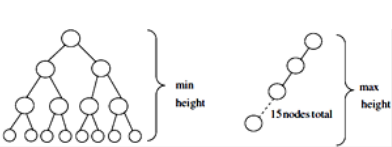# GATE Solved Paper 2017-19 - GATE 2017 Shift 1

>>>>>>>>GATE 2017 Shift 1

• A

log2n, 100/n, 10, √n, n• B

100/n, 10, log2n, √n, n• C

10, 100/n, √n, log2n, n• D

100/n, log2n, 10, √n, n• Option : B
• Explanation :
As n → ∞, 100/n < 10 < log2n < √n < n

• A

4 and 15 respectively• B

3 and 14 respectively• C

4 and 14 respectively• D

3 and 15 respectively• Option : B
• Explanation :Min height = floor(log2N) = floor(log215)=3
Max height n-1 = 14, when the tree is either left skewed or right skewed.

• Option : D
• Explanation :
A) is wrong. We don’t need to cast the result as void * is automatically and safely promoted to any other pointer type in this case.
B) It is discarded for obvious reason.
C) is wrong, because dangling pointer is nothing but the pointer which is pointing existing memory (deallocated or deleted memory) which is not happening here.
D) is the answer. When you are calling malloc second time, new location is assigned to x and previous memory location is lost and now we don’t have no reference to that location resulting in memory leak.

• Option : B
• Explanation :  CLK Q1 Q0 0 1 1 1 0 1 2 1 0 3 1 1 4 0 1

After 3rd clock pulse :11
After 4th clock pulse: 01
Qt can be observed from the diagram that :
Q0 Q1 after the 3rd cycle are 11 and after the 4th cycle are 01
Therefore, option B is correct

• A

Return of 6 and 6 respectively.• B

Infinite loop and abnormal termination respectively.• C

Abnormal termination and infinite loop respectively.• D

Both terminating abnormally• Option : C
• Explanation :
In given function foo every time in the while foo is called value 3 because val is passed with post decrement operator so the value 3 is passed and val is decremented later. Every time the function is called a new variable is created as the passed variable is passed by value, with the value 3. So the function will close abruptly without returning any value.
In the function bar, in the while loop value the value of val variable is not decrementing, it remains 3 only. Bar function in the while loop is called with val-1 i.e 2 but value of val is not decremented, so it will result in infinite loop.
Related Quiz.
GATE 2017 Shift 1